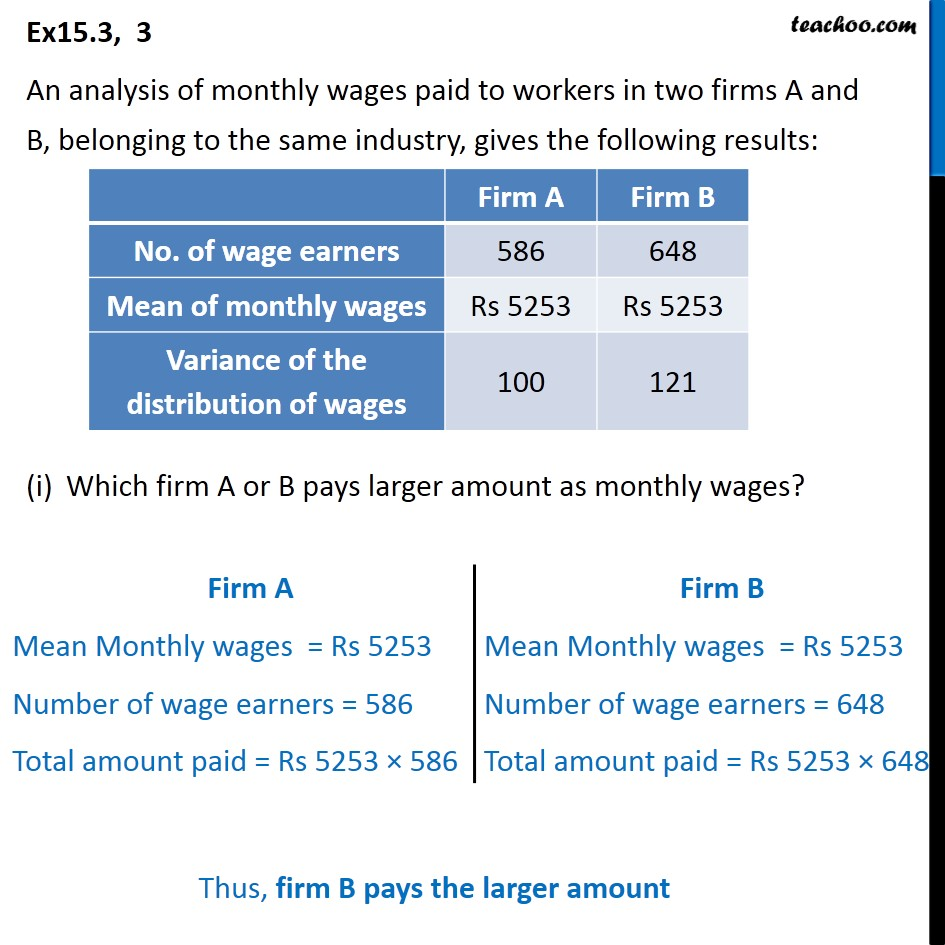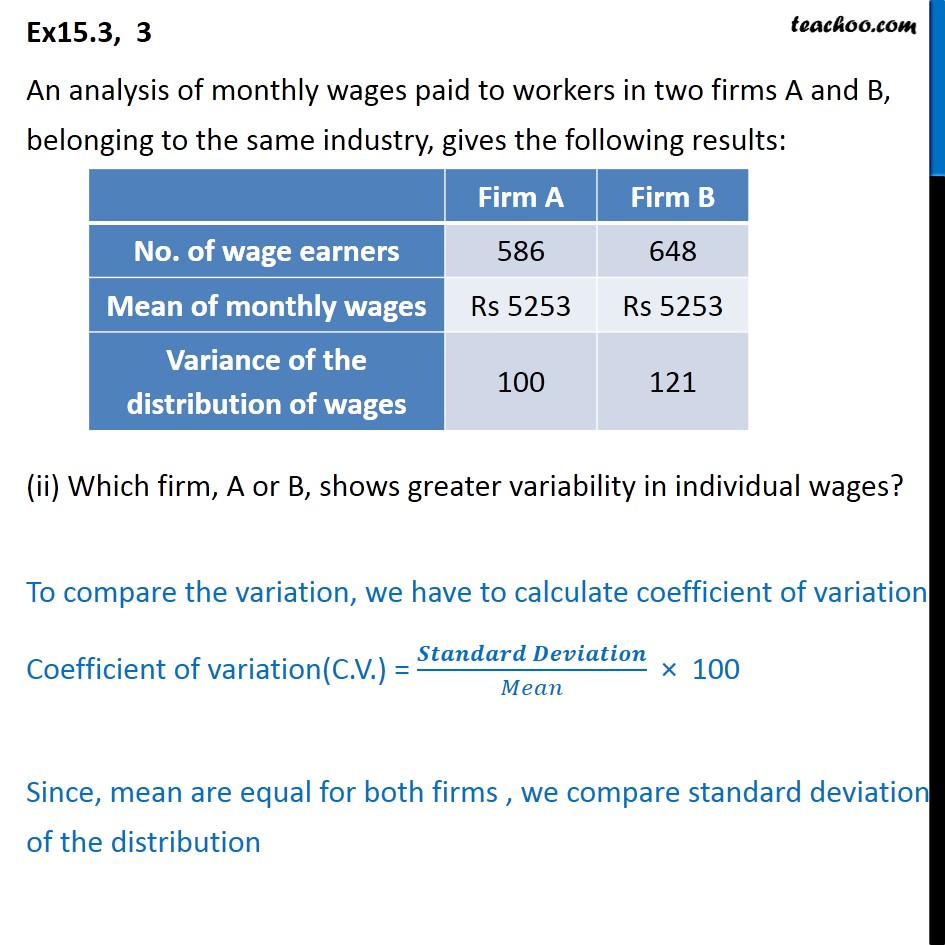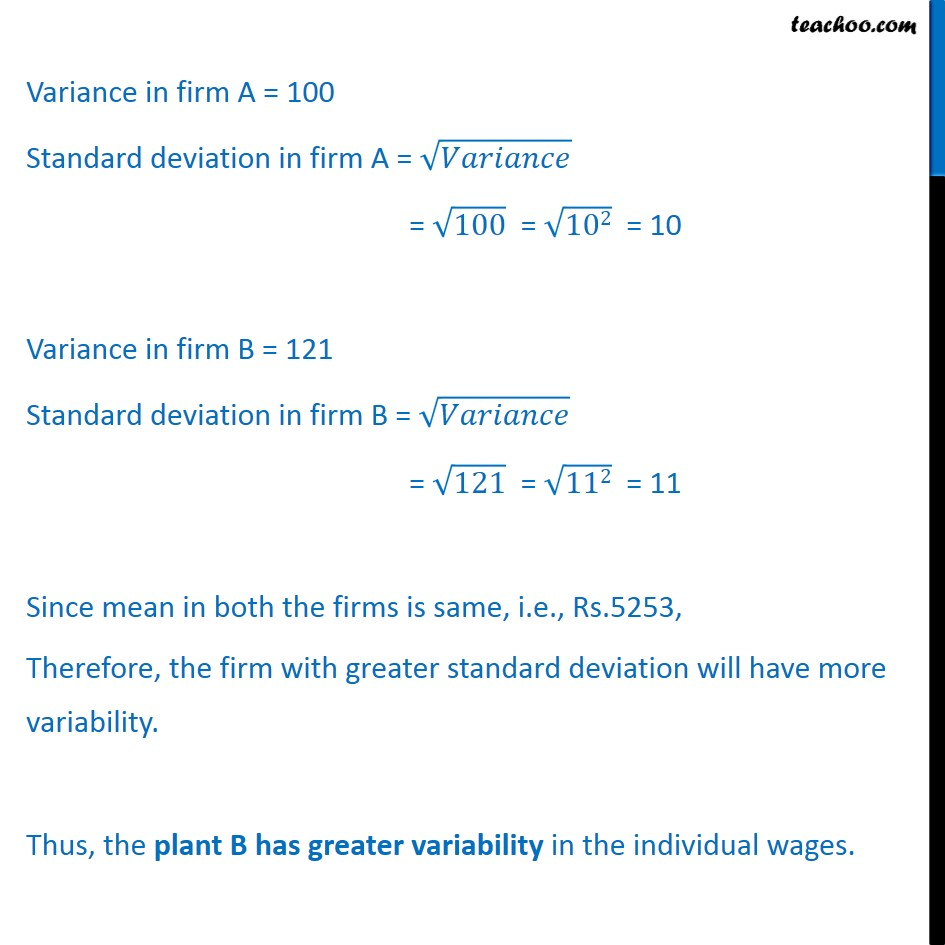Coefficient of Variation

Chapter 13 Class 11 Statistics
Serial order wiseLearn in your speed, with individual attention - Teachoo Maths 1-on-1 Class

### Transcript

Ex15.3, 3 An analysis of monthly wages paid to workers in two firms A and B, belonging to the same industry, gives the following results: Which firm A or B pays larger amount as monthly wages? Ex15.3, 3 An analysis of monthly wages paid to workers in two firms A and B, belonging to the same industry, gives the following results: (ii) Which firm, A or B, shows greater variability in individual wages? Variance in firm A = 100 Standard deviation in firm A = = 100 = 102 = 10 Variance in firm B = 121 Standard deviation in firm B = = 121 = 112 = 11 Since mean in both the firms is same, i.e., Rs.5253, Therefore, the firm with greater standard deviation will have more variability. Thus, the plant B has greater variability in the individual wages.